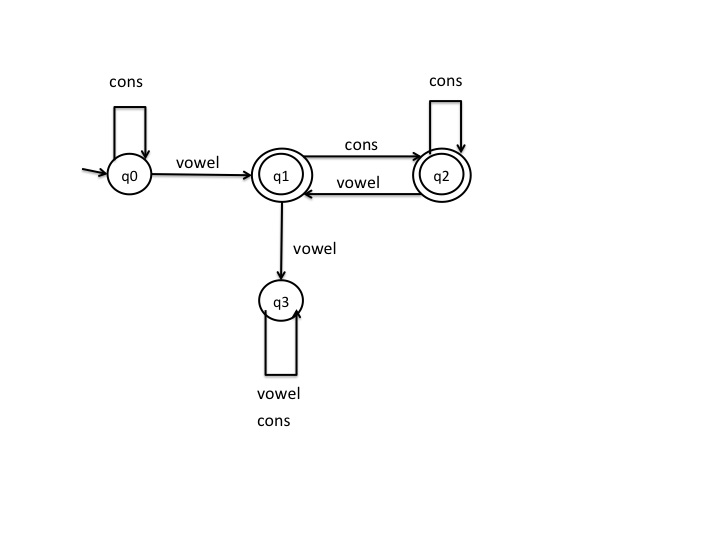# Midterm Exam Solution

CS 536, Spring 2013

## Question 1 (12 points)

### Part (a) (6 points)

There are many possible ways to do this; here's one:### Part (b) (6 points)

There are many possible ways to do this; here are two:

• cons* vowel (cons+ vowel)* cons*

• cons* vowel (cons vowel | cons+)*

## Question 2 (10 points)

```stmt ::= FOR LPAREN forCond RPAREN LCURLY varDeclList stmtList RCURLY
forCond ::= ID ASSIGN exp COLON exp optFor
optFor ::= COLON exp
|  /* epsilon */
```

## Question 3 (27 points)

### Part (a) (15 points)

```exp → exp ? term  |  term

term → factor \$ term  |  factor

factor → INTLIT  |  ( exp )
```

### Part (b) (4 points)

```        expr
/  |  \
/    |    \
expr     ?    term
|           /  |  \
term    factor  \$  term
|        |         |
factor   INTLIT    factor
|       (2)     /  |   \
INTLIT           (  expr  )
(1)               / |  \
expr ?  term
|       |
term   factor
|       |
factor  INTLIT
|      (4)
INTLIT
(3)
```

### Part (c) (4 points)

```exp → term exp'

exp' → ? term exp'  |  ε

term → factor \$ term  |  factor

factor → INTLIT  |  ( exp )
```

### Part (d) (4 points)

```exp → term exp'

exp' → ? term exp'  |  ε

term → factor term'

term' → \$ term  |  ε

factor → INTLIT  |  ( exp )
```

## Question 4 (15 points)

Grammar ruleTranslation rule
program → varDeclList stmtListprogram.trans = stmtList.trans
varDeclList → varDeclList varDecl
varDeclList → varDecl
varDecl → type ID ;
type → INT
type → BOOL
stmtList → stmtList stmtstmtList1.trans =
union(stmtList2.trans, stmt.trans)
stmtList → stmtstmtList.trans = stmt.trans
stmt → ID = exp ;stmt.trans = exp.trans
stmt → IF ( exp ) { varDeclList stmtList }stmt.trans =
union(exp.trans, stmtList.trans)
exp → IDexp.trans = new List(ID.val)
exp → INTLITexp.trans = new List()
exp → exp + expexp1.trans =
union(exp2.trans, exp3.trans)
exp → exp * expexp1.trans =
union(exp2.trans, exp3.trans)
exp → exp == expexp1.trans =
union(exp2.trans, exp3.trans)

## Question 5 (36 points)

### Part (a) (24 points)

Non-terminal XFIRST(X)FOLLOW(X)
block LCURLY EOF, ELSE, ID, IF, RETURN, RCURLY
declList TYPE, ε ID, IF, RETURN, RCURLY
stmtList ID, IF, RETURN, ε RCURLY
decl TYPE TYPE, ID, IF, RETURN, RCURLY
stmt ID, IF, RETURN ID, IF, RETURN, RCURLY
exp ID, INT SEMI, RPAREN

### Part (b) (12 points)

Snapshot 1:

 current stack ``` stmtList RCURLY EOF```

Snapshot 2:

partial parse tree

```               block
__/ || \__
_____/ __/  \__ \_____
/      /        \      \
LCURLY  declList  stmtList  RCURLY
/   \
/     \
decl  declList
/  | \
/   |  \
TYPE ID  SEMI
```

Snapshot 3:

input: LCURLY RCURLY

 current stack ``` stmtList RCURLY EOF```